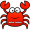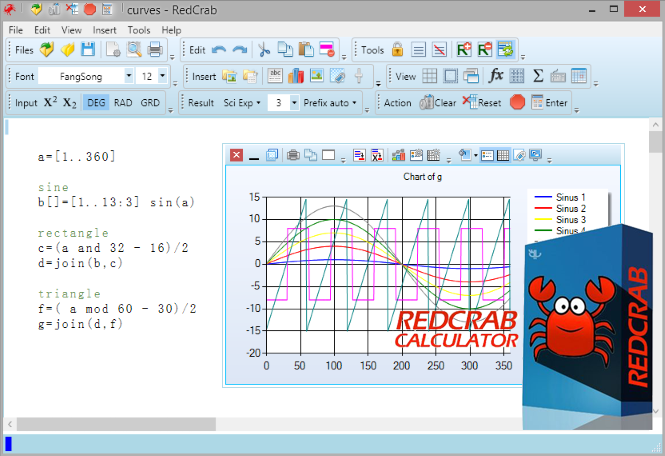#RedCrab Calculator PLUS7.15.0.736

## Free Download RedCrab Calculator PLUS - Fullscreen editor for the free placement of algebraic formulas on the worksheet in mathematical notation

### Overview of RedCrab Calculator PLUS Mathematics software:RedCrab Calculator is a mathematics software with fullscreen editor for the free placement of algebraic formulas on the worksheet in mathematical notation. Results can be displayed in different numeric formats and in diagrams. Images and texts can be inserted.

### Overview of RedCrab Calculator 7 Features:

• Free placement of mathematical formulas in natural notation
• Numerics formats of results
• Decimal fixed point, floating point, exponent
• Date and time format
• Optionally with text
• With units of measurement
• With prefix
• Individual assignment of output formats to variables
• Audible announcement of the results
• Number of chart types for graphical display of the results
• 2D function plotter
• Calculating with units of measure. Automatic conversion of different systems in a formula (km, miles, acres, liter, yard). A
• Defining your own mathematical functions
• Accuracy digits: 14 / 28
• Print selected areas of the worksheet
• Insert of text boxes
• Integrated functions: >250
• Interface (API) to perform its own functions in external programs
• Slider for variable data input.

### RedCrab PLUS:

The shareware RedCrab PLUS extends the range of functions with additional functions for statistics, finances, matrices and special applications, as well as calculating with complex numbers and units of measure. Plotting of 2D graphics and charts.

### System Requirements:

• Supported OS: Windows 7/8/10
• Processor: Pentium IV or above
• RAM: 1 GB RAM
• Free Hard Disk Space: 20 MB or more.
• Evaluate:
• Operating system:
• CalibreE-book library management